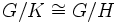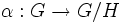# Prime index and quotient-subisomorph-containing implies index-unique

## Statement

Suppose$G$ is a group, and$H$ is a Subgroup of prime index (?) (specifically, a Normal subgroup of prime index (?)) that is also a Quotient-subisomorph-containing subgroup (?). Then,$H$ is an Index-unique subgroup (?): there is no other subgroup of$G$ with the same index.

When$G$ is a finite group, this is equivalent to saying that$H$ is an order-unique subgroup: there is no other subgroup of$G$ of the same order.

## Proof

Given: A group$G$, a prime number$p$, a subgroup$H$ of prime index in$G$ such that$H$ is contained in the kernel of any homomorphism from$G$ to$G/H$.

To prove:$H$ is the only subgroup of index$p$ in$G$.

Proof: Suppose$K$ is a subgroup of index$p$. Then,$G/K \cong G/H$. Thus, the quotient map$G \to G/K$ can be composed with this isomorphism, giving a map$\alpha:G \to G/H$ with kernel$K$. Since$H$ is quotient-homomorph-containing in$G$, this implies$H \le K$. But since both have index$p$, this forces$H = K$.